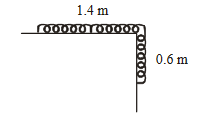# Q.> A uniform chain of length 2 m is kept on a table such that a length of  60 cm hangs freely from the edge of the table. The total mass of the chain is 4 kg . What is the work (in Joule) done in pulling the entire chain on the table ?My answer:mass per unit centimetre:4000/200=20gdisplacement=60cmtotal mass of 60cm chain=1200gwe know: W=F.sF=m.g=1200gm*10m/s=1.2kg*10m/ss=60cm=0.6mw=F.s=1.2*10*0.6=7.2Jso total work done is 7.2J

You have done it wrong.

As we learnt in

Definition of work done by variable force -

- wherein

is variable force and  is small displacementConsider a small part dx at a depth x from table.

Work done in lifting this small portion is dw = dm gx

Total work done

### Preparation Products

##### JEE Main Rank Booster 2021

This course will help student to be better prepared and study in the right direction for JEE Main..

₹ 13999/- ₹ 9999/-
##### Knockout JEE Main April 2021 (Subscription)

An exhaustive E-learning program for the complete preparation of JEE Main..

₹ 6999/- ₹ 5/-
##### Knockout JEE Main April 2021

An exhaustive E-learning program for the complete preparation of JEE Main..

₹ 22999/- ₹ 14999/-
##### Knockout JEE Main April 2022

An exhaustive E-learning program for the complete preparation of JEE Main..

₹ 34999/- ₹ 24999/-